#Comparison of VE saturation and height formulations

Here, we study the use of different formulations for the vertical equlibrium approximations to the two-phase flow in reservoirs.

1. Using the heightof the CO2 column as primary variable, a mimetic discretization of the pressure equation, and a transport solver based on explicit time stepping. This was the original formulation in the vertical average module.
2. Using fractional height (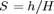) as primary variable, a standard two-point discretization for the pressure equation, and the standard implicit transport solver from mrst core.

The example also sets up a complete input deck (in Eclipse format) which can be used for simulation by traditional solvers

## Background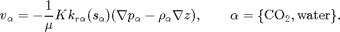By integrating in the vertical direction and assuming vertical equlibrium with a sharp interface between the two fluids and constant permeability and porosity, we obtain the upscaled version of Darcy's law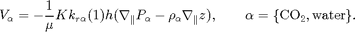If we now introduce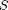as the fractional height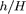of the CO2 column, Darcy's law can be reformulated usingas variable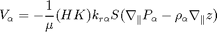As usual the pressure equation from incompressible flow is obtaind by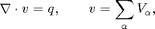whereis the Darcy velocity (total velocity) and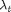is the total mobility, which depends on the water saturation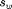.

The transport equation (conservation of the CO2 phase) in its simplest form for the S-formulation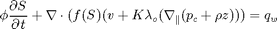and for theformulation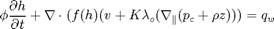Notice, however, that the fractional flow functionsand the capillary pressure function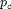have a different interpretation than in a standard fractional flow model.

try
require deckformat gridtools
catch %#ok<CTCH>
end


## Parameters for the simulation

gravity on
[nx,ny,nz] = deal(50, 1, 1);     % Cells in Cartsian grid
[Lx,Ly,H]  = deal(2000,1000,15); % Physical dimensions of reservoir
total_time = 100*year;           % Total simulation time
nsteps     = 40;                 % Number of time steps in simulation
dt         = total_time/nsteps;  % Time step length
inj_time   = total_time/10;      % Injetection time
perm       = 100;                % Permeability in milli darcies
K          = perm*milli*darcy(); % Permeability in SI units
phi        = 0.1;                % Porosity
depth      = 1000;               % Initial depth
ipress     = 200;                % Initial pressure


## Create input deck and convert to SI units

Create an input deck that can be used together with standard black-oil solvers. To this end, the 2D reservoir is described as a volumeric slice of a 3D reservoir.

deck = sinusDeck([nx ny nz], [Lx Ly H], nsteps, dt, -pi/180, depth, phi, ...
perm, (H*phi*Lx*Ly)*0.2*day/inj_time, ipress);
deck = convertDeckUnits(deck);


## Instantiate grid and rock structures

Read the input deck to create a 3D grid, from which we can construct the top-surface grid used for the vertical equilibrium calculations

G = initEclipseGrid(deck);
G = computeGeometry(G);

Gts = topSurfaceGrid(G);
Gts.cells.H = H*ones(Gts.cells.num,1);
Gts.columns.dz = ones(numel(Gts.columns.cells),1)*H/nz;
Gts.columns.z = cumulativeHeight(Gts);

% get permeability
rock   = initEclipseRock(deck);
rock2d = averageRock(rock, Gts);

% Note that the top-surface grid is modified with an extra field that
% defines a function handle to how to calculate the gravity contribution.
% This is the part which is VE spesific.
Gts.grav_pressure

ans =

@(g,omega)gravPressureVE_s(g,omega)



## Define boundary conditions, source terms, and fluid properties

Boundary conditions are no-flow and there are no source terms. Hence, these need not be specified explicitly, but we include them as a hook if the reader wants to extend the routine to more general cases.

[bc_s, bc_h]   = deal([]);
[src_s, src_h] = deal([]);
for i=1:numel(src_h)
src_h.sat = nan;
src_h.h = Gts.cells.H(src_s.cell);
end

% Extract fluid properties from input deck, but use different saturations.
mu = [deck.PROPS.PVDO{1}(1,3), deck.PROPS.PVTW(1,4)];
rho = deck.PROPS.DENSITY(1:2);
sr = 0.3;
sw = 0.3;


## Define wells

We extract arbitrarily the first set of wells from the deck constructed for a general black-oil solver. Since the grid specified in the input deck is only one cell thick, the well indices calculated in 3D will also be correct for the VE simulation. However, we need to make to other modifications. First, if the well is specified as 'resv', we change the specification to rate. Likewise, we correct the definition of input value according to solver so that CO2 is injected correctly in each two-phase VE solver.

W_3D = processWells(G, rock, deck.SCHEDULE.control(1));
for i=1:numel(W_3D)
if(strcmp(W_3D(i).type,'resv'))
W_3D(i).type='rate';
end
end
W_h = convertwellsVE(W_3D, G, Gts, rock2d,'ip_simple');
W_s = convertwellsVE(W_3D, G, Gts, rock2d,'ip_tpf');
for i=1:numel(W_h)
W_s(i).compi=[1 0];
W_h(i).compi=nan;
W_h(i).h = Gts.cells.H(W_h(i).cells);
end


## Set up solver based on h-formulation

Create a cell array to hold helper variables and function handles for each solver.

problem = cell(2,1);
tmp.fluid = initVEFluidHForm(Gts, 'mu', mu, 'rho', rho, 'sr', sr,'sw', sw);
tmp.sol   = initResSol(Gts, 0);

% Set up wells, boundary conditions and source terms
[tmp.W, tmp.bc, tmp.src] = deal(W_h, bc_h, src_h);

% Define pressure and transport solvers with common interfaces. For the
% mimetic pressure solver, we need to precompute inner products
S = computeMimeticIPVE(Gts,rock2d,'Innerproduct','ip_simple');
tmp.psolver = @(sol, fluid, W, bc, src) ...
solveIncompFlowVE(sol, Gts, S, rock2d, fluid, 'wells', W, 'bc', bc, 'src', src);
tmp.tsolver = @(sol,fluid, dt, W, bc, src) ...
explicitTransportVE(sol, Gts, dt, rock, fluid, 'computeDt', true, ...
'intVert_poro', false,'intVert',false,'wells',W,'bc',bc, 'src', src);

% As this solver solves for height of CO2 plume, it is not neccessary to
% compute h from saturation values but have to find the saturation
tmp.compute_h=false;
tmp.compute_sat=true;

% Miscellaneous
tmp.col  ='r-';
tmp.name = 'H formulation using explicit transport and mimetic discretization';

% Insert in cell array
problem{1} = tmp;
clear tmp


## Set up solver based on S-formulation

The S-formulation uses standard solvers, except for the function handle 'twophaseJacobian' in the solution object, which here points to a slightly extended version of the original formulation of the Jacobi system ('twophaseJacobian') normally used in MRST

tmp.fluid = initSimpleVEFluid_s('mu', mu, 'rho', rho, ...
'height', Gts.cells.H, 'sr', [sr, sw]);
tmp.sol   = initResSolVE_s(Gts, 0, [1 0]);
tmp.sol.twophaseJacobian

% Set up wells, boundary conditions and source terms
[tmp.W, tmp.bc, tmp.src] = deal(W_s, bc_s, src_s);

% For the TPFA pressure solver, we need to calculate transmissibilities for
% the 2D top-surface grid. Depending on the definition of area and length
% in the top-surface grid this may or may not treat length along the
% surface.
T = computeTrans(Gts,rock2d);
T = T.*Gts.cells.H(gridCellNo(Gts));
tmp.psolver = @(sol,fluid, W, bc, src) ...
incompTPFA(sol, Gts, T, fluid, 'wells', W, 'bc', bc, 'src', src);
tmp.tsolver = @(sol,fluid,dt,W,bc, src) ...
implicitTransport(sol, Gts, dt, rock2d, fluid, 'wells', W, 'bc', bc, 'src', src);

% One need to calculate h separatly since the solver does not
tmp.compute_h = true;
tmp.compute_sat = false;

% Miscellaneous
tmp.col = 'gs';
tmp.name = 'S formulation using implicit transport and two point flux';

% Insert in cell array
problem{2} = tmp;
clear tmp;

ans =

@twophaseJacobianWithVE_s



## Initialize solution and variables

for kk=1:numel(problem)
s0 = deck.SOLUTION.SOIL;
h0 = deck.SOLUTION.SOIL.*Gts.cells.H;
problem{kk}.sol.s = s0;
problem{kk}.sol.h = h0;
problem{kk}.sol.extSat = [s0, s0];
problem{kk}.sol.h_max = h0;

% Timers for pressure and transport solve
problem{kk}.p_time=0;
problem{kk}.t_time=0;
end

% Variables used for plotting
xc = Gts.cells.centroids(:,1);
zt = Gts.cells.z;
zb = Gts.cells.H+zt;
vol  = rock2d.poro.*Gts.cells.volumes.*Gts.cells.H;


## Run the transport simulation.

fig1 = figure(1); p=get(fig1,'Position'); set(fig1,'Position', [p(1:2) 1020 420]);
fig2 = figure(2);

fprintf(1,' Free volumes [1e6 m^3]\n');
fprintf(1,'Time     H-form     S-form\n');
fprintf(1,'------------------------------\n');
fprintf(1,'%6.2f   %f   %f\n', 0.0, 0.0, 0.0);
t = 0;
while t < total_time
set(0, 'CurrentFigure', fig1); clf
set(0, 'CurrentFigure', fig2); clf
for kk=1:numel(problem)
tmp = problem{kk};

if(t<inj_time)
[W, bc, src] = deal(tmp.W, tmp.bc, tmp.src);
else
[W, bc, src] = deal([]);
end

% Solve the pressure
timer = tic;
tmp.sol = tmp.psolver(tmp.sol, tmp.fluid,  W, bc, src);
tmp.p_time = tmp.p_time + toc(timer);

% Using the computed fluxes, solve the transport
timer = tic;
if t>inj_time
% uncomment to forcing zero total flow
tmp.sol.flux(:) = 0;
end
tmp.sol = tmp.tsolver(tmp.sol,tmp.fluid,dt,W,bc, src);
tmp.t_time =tmp.t_time + toc(timer);

if tmp.compute_h
% Compute h if this problem needs it
[h, h_max]    = tmp.fluid.sat2height(tmp.sol);
tmp.sol.h     = h;
tmp.sol.h_max = h_max;

% set the value s_max for convenience
tmp.sol.s_max = tmp.sol.extSat(:,2);
end
if tmp.compute_sat
% if the solver does not compute s and s_max, calculate them
h     = tmp.sol.h;
h_max = tmp.sol.h_max;
tmp.sol.s =  (h*(1-sw) + (h_max - h)*sr)./Gts.cells.H;
tmp.sol.s_max =  h_max*(1 - sw)./Gts.cells.H;
end

% Special type of plot if solving a 2D VE problem
if Gts.cartDims(2)>1
subplot(numel(problem),2,(2*(kk-1))+1)
pcolor(X,Y,reshape(tmp.sol.h,Gts.cartDims))
if kk==1, cxs=caxis(); else caxis(cxs); end;
title(['Height ',num2str(kk)]);

subplot(numel(problem),2,(2*(kk-1))+2)
pcolor(X,Y,reshape(tmp.sol.max_h,Gts.cartDims))
caxis(cxs)

problem{kk} = tmp;
continue;
end

% Plot current and observed maximal values for h and S
set(0, 'CurrentFigure', fig1);
subplot(2,4,1), hold on
plot(xc, tmp.sol.h, tmp.col); ylim([0 H])
if kk==1, axh=axis(); else axis(axh); end
title('Height'); xlabel('x'); ylabel('h');

subplot(2,4,2), hold on
plot(xc, tmp.sol.h_max, tmp.col); ylim([0 H])
if kk==1, axh_max=axis(); else axis(axh_max);  end
title('Max height'); xlabel('x'); ylabel('h_max') ;

subplot(2,4,5), hold on
plot(xc, tmp.sol.s(:,1), tmp.col); ylim([0 1])
if kk==1; axs=axis(); else axis(axs); end
title('Saturation'); xlabel('x'); ylabel('s')

subplot(2,4,6), hold on
plot(xc, tmp.sol.s_max(:,1), tmp.col); ylim([0 1]);
if kk==1, axs_max=axis(); else axis(axs_max); end
title('Max Saturation'); xlabel('x'); ylabel('s_max')

set(0, 'CurrentFigure', fig1);
subplot(2,4,[3 4 7 8]); set(gca,'YDir','reverse')
hold on
plot(xc,zt+tmp.sol.h, [tmp.col(1) '-'])
plot(xc,zt+tmp.sol.h_max, [tmp.col(1), '--'])
if kk==2
patch(xc([1 1:end end]), [min(zt)-1; zt; min(zt)-1], .85*[1 1 1]);
patch(xc([1 1:end end]), [max(zb)+1; zb; max(zb)+1], .85*[1 1 1]);
legend('Free CO2, h', 'Max CO2, h', 'Free CO2, S', 'Max CO2, S', 4);
box on;axis tight; title('Surfaces'); xlabel('x'); ylabel('depth')
end

% Plot pressures.
set(0, 'CurrentFigure', fig2); set(gca, 'YDir', 'reverse')
hold on
plot(xc, tmp.sol.pressure/barsa, tmp.col)
if ~tmp.compute_sat
% Also plot CO2 pressure: The default is to use the pressure of
% the second phase pressure for the incompressible solvers
plot(xc,(tmp.sol.pressure-tmp.fluid.pc(tmp.sol)),['-',tmp.col])
else
plot(xc,(tmp.sol.pressure-norm(gravity)*rho(1)*tmp.sol.h),['s',tmp.col])
end
xlabel('x'); ylabel('pressure')
title('Comparing pressure for the different solvers')

% Update solution object
problem{kk} = tmp;
end
set(0, 'CurrentFigure', fig1); drawnow;
set(0, 'CurrentFigure', fig2); drawnow;

t=t+dt;

% Print CO2 inventory
fprintf(1,'%6.2f   %f   %f\n', t/year, ...
sum(problem{1}.sol.s.*vol)/1e6, ...
sum(problem{2}.sol.s.*vol)/1e6 );

end
fprintf(1,'-----------------------------------------\n\n');

 Free volumes [1e6 m^3]
Time     H-form     S-form
------------------------------
0.00   0.000000   0.000000
2.50   0.150000   0.148380
5.00   0.299427   0.288994
7.50   0.431549   0.403057
10.00   0.536992   0.505008
12.50   0.536992   0.505008
15.00   0.536992   0.505008
17.50   0.536992   0.505008
20.00   0.536992   0.505008
22.50   0.536992   0.505008
25.00   0.536992   0.505008
27.50   0.536992   0.505008
30.00   0.536992   0.505008
32.50   0.536992   0.505008
35.00   0.536992   0.505008
37.50   0.536992   0.505008
40.00   0.536992   0.505008
42.50   0.536992   0.505008
45.00   0.536992   0.505008
47.50   0.536992   0.505008
50.00   0.536992   0.505008
52.50   0.536992   0.505008
55.00   0.536992   0.505008
57.50   0.536992   0.505008
60.00   0.536992   0.505008
62.50   0.536992   0.505008
65.00   0.536992   0.505008
67.50   0.536992   0.505008
70.00   0.536992   0.505008
72.50   0.536992   0.505008
75.00   0.536992   0.505008
77.50   0.536992   0.505008
80.00   0.536992   0.505008
82.50   0.536992   0.505008
85.00   0.536992   0.505008
87.50   0.536992   0.505008
90.00   0.536992   0.505008
92.50   0.536992   0.505008
95.00   0.536992   0.505008
97.50   0.536992   0.505008
100.00   0.536992   0.505008
-----------------------------------------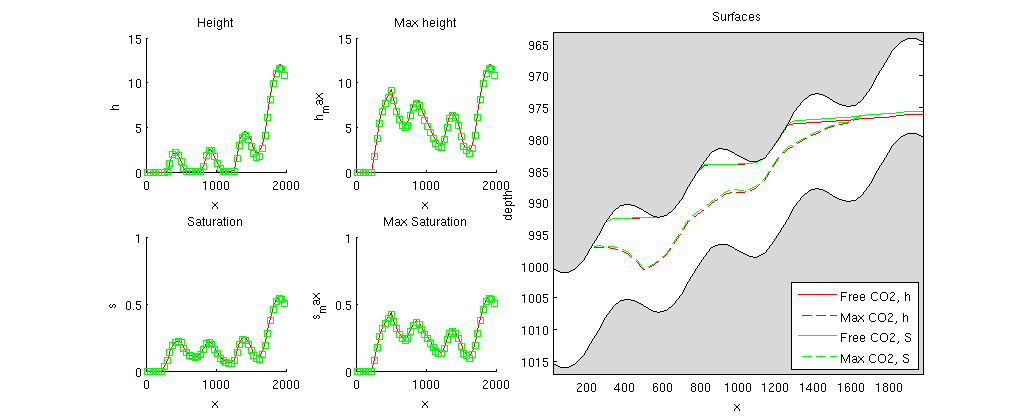## Output calculation time

for kk = 1:numel(problem)
fprintf('Time used for solving with:\n\t%s\n',problem{kk}.name);
fprintf('\tPressure time : %2.2d sec\n' ,problem{kk}.p_time)
fprintf('\tTransport time : %2.2s sec\n',problem{kk}.t_time)
end

Time used for solving with:
H formulation using explicit transport and mimetic discretization
Pressure time : 1.88e-01 sec
Transport time : 1.08e+00 sec
Time used for solving with:
S formulation using implicit transport and two point flux
Pressure time : 1.46e-01 sec
Transport time : 1.22e+01 sec


## Compare with analytical pressure

The state is almost stationary and we therefore add a comparison with pressures computed analytically. In the example, it is always only water at the bottom. For hydrostatic conditions, we can therefore find the pressure at the bottom relative to a given datum (here, we use the pressure at the top of the first column).

set(0, 'CurrentFigure', fig2); clf, hold on
set(gca, 'YDir', 'reverse')

p   = tmp.sol.pressure(1);
hh  = problem{1}.sol.h;
hs  = problem{2}.sol.h;
rwg = rho(2)*norm(gravity);
z   = Gts.cells.z;
H   = Gts.cells.H;

% Water pressure at the bottom
pwb = p + rwg*(z - z(1) + H(1));
plot(xc, pwb,'dk-')
text(xc(6),pwb(5), '\leftarrow Water pressure at bottom', ...
'HorizontalAlignment', 'left')

% Water pressure extrapolated hydrostatic to the top of the reservoir
pwt = p + rwg*(z - z(1));
plot(xc, pwt, 'dk-')
text(xc(end-4), pwt(end-3), 'Water extrapolated to top \rightarrow', ...
'HorizontalAlignment', 'right')

% water pressure at interface between CO2 and water
pwi = p + rwg*(z - z(1) + hh);
plot(xc, pwi,'dk-')
text(xc(10),pwi(7),'\uparrow Pressure at interface')

% CO2 pressure at the top surface
pco2 = p + rwg*(z+-z(1)+H(1)) - norm(gravity)*(rho(2)*(H - hs) + rho(1)*hs);
plot(xc, pco2, 'dr-');
text(xc(end-4), pco2(end-3),'Pressure at top \rightarrow', ...
'HorizontalAlignment', 'right', 'Color', 'r')

% In this plot of the pressure at the end we see
%    - The mimetic calculate the pressure at the interface between
%        CO2 and water
%      - The tpfa with the given fluid calculate the extrapolated
%        water pressure at the top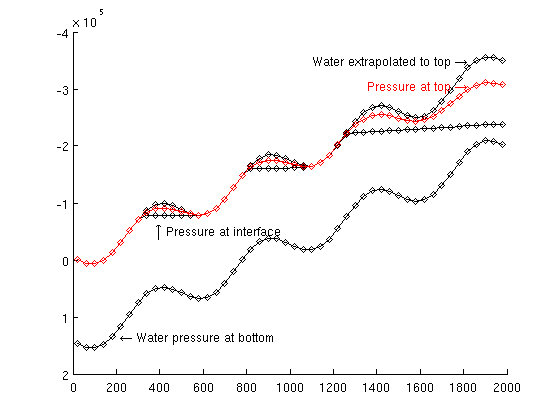Published February 12, 2013